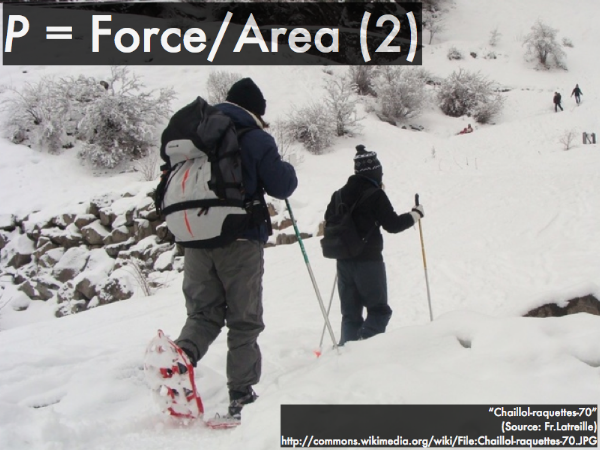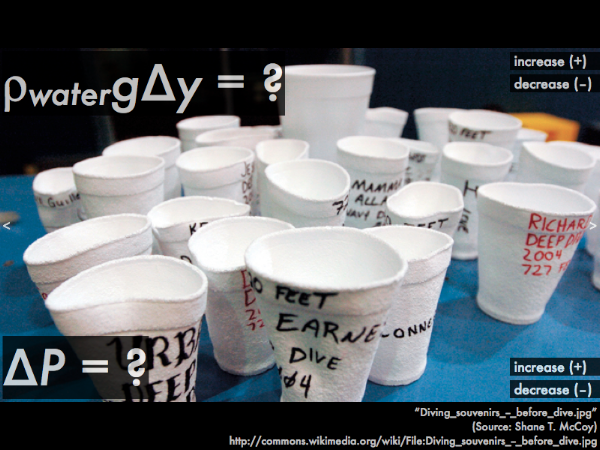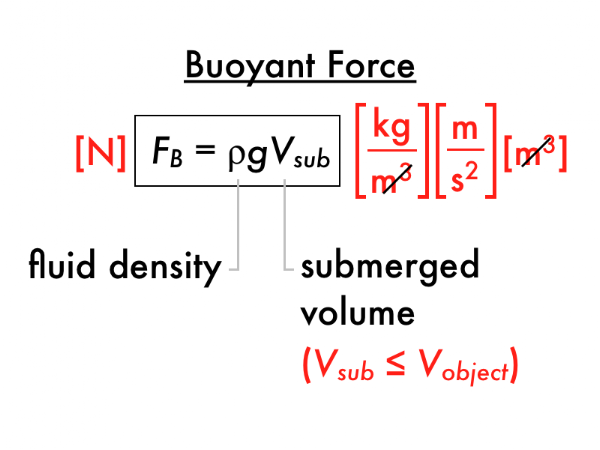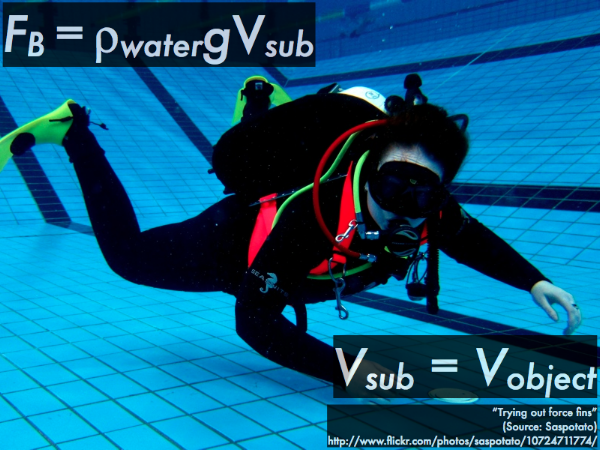## 20141025

### Physics presentation: static fluidsIn this scene from Man from Atlantis, Mark Harris (played by Patrick Duffy) is put into a water pressure chamber that simulates different depths, and watches as test canisters are crushed by pressures equivalent to 20,000 ft, 25,000 ft, and 30,000 ft below sea level.On a more reality-based aside, consider tourists who float in the high salinity waters of the Dead Sea.We'll discuss these two different aspects of static fluids here: pressure, and buoyancy.First, pressure--as a force per unit area density, then reinterpreted as a pressure per unit area density.Pressure can be consider as the amount of force exerted over a certain area on a surface, whether by a macroscopic object, or by the random bombardment by atoms/molecules of gases or liquids. If you don't wear snowshoes, the force of your weight, distributed over the area of your feet will create a pressure that cannot be supported by soft, unpacked snow, and your feet will sink in.However, the force of your weight distributed over a much larger snowshoe area will reduce the pressure exerted on the snow, and you will not sink in much, if at all.From the definition of pressure as a force per area density, the unit of pressure is pascals (Pa), equivalent to N/m2.

A more useful interpretation of pressure, especially in regards to fluids (gases and liquids) is to think of it as an energy per unit volume. Notice how the units of pascals equals N/m2, and when both numerator and denominator by are multiplied by meters (m), these units become N/m2 = (N·m)/(m3) = J/m3.By interpreting pressure as a form of energy per unit volume, we can connect it to gravitational potential energy per unit volumeUgrav/V = m·g·y/V = (m/Vg·∆y = ρ·g·∆y, which also has units of J/m3.

Pressure and gravitational potential energy per unit volume are then terms in an energy density "conservation" equation, and they are allowed to "exchange" Pa provided the fluid is static and there is no external work being put in or taken out of the fluid. In this form, then by picking two locations in the same static fluid, an increase or decrease in the ρ·g·∆y must have a corresponding decrease or increase in pressure (∆P).A weather balloon that is partially filled at ground level will rise, and will seemingly inflate and eventually explode at very high elevations.

To explain what's going on here, we are going to analyze the static fluid that exists at both ground level and at a higher elevation: the air surrounding the balloon (i.e., the entire atmosphere), and not the contents of the balloon, which do not simultaneously exist at both locations (as it is "transported," and technically not a "static" fluid.)

Let's compare the air surrounding the balloon at ground level, and compare it to the air at the final higher elevation. Since the gravitational potential energy density depends on elevation, as the elevation of the balloon increases, the gravitational potential energy density of the surrounding air increases.

Looking at the energy density "conservation" equation for static fluids:

0 = ΔP + ρ·g·∆y,

Since the gravitational potential energy density of the air surrounding the balloon increases as it moves to higher elevations, then ρ·g·∆y is positive. In order to balance out this equation to equal zero on the left-hand side, the pressure of the air surrounding the balloon must have a corresponding decrease, making ΔP negative, such that:

0 = (–) + (+),

meaning that there is more air pressure at ground level than at a higher elevation. Essentially the pressure within the balloon remains constant, and because it is surrounded with lower pressure air at a higher elevation, the balloon will expand in size, and eventually "pop."Notice the full-sized Styrofoam™ cup in the back, compared to other cups that were carried in the outside storage compartment of a submarine, where the air pockets inside these cups were collapsed by the surrounding water, effectively permanently shrinking the sizes of these cups. During this process, did the ρ·g·∆y increase or decrease? Did the water pressure surrounding the cups increase or decrease?Second, buoyancy.The buoyant force on an object depends on the density ρ of the fluid, and the volume of the object that is actually submerged in the fluid. While this is a simple definition, knowing the appropriate amount of volume to use in this equation is key to understanding buoyancy.For a fully-submerged object, the volume used in calculating the buoyant force is the volume of the entire object, such that the buoyant force is given by:

FB = ρ·g·V,

where ρ is the density of the surrounding fluid (water), and volume V is the entire volume of the diver, as he is fully submerged.

Here, since the object (the submerged diver) is floating underwater, Newton's first law applies, and the downwards weight force and the upwards buoyant force balance out.For a partially-submerged object like this red ship, the volume used in calculating the buoyant force is not the volume of the entire object, but only the portion of the object that is actually submerged.

For the red ship, which Newton's law applies to its motion? How do the magnitudes of the downwards weight force and the upwards buoyant force compare? What fluid density should be put into the ρ in the buoyant force calculation?